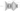← Go BackMarch 22, 20203 min read • IN tech

## Neural Net Problems - Exercise 2

Here are my solutions to exercise 2.

## The Architecture of Neural Networks

### Question

There is a way of determining the bitwise representation of a digit by adding an extra layer to the three-layer network above. The extra layer converts the output from the previous layer into a binary representation, as illustrated in the figure below. Find a set of weights and biases for the new output layer. Assume that the first $3$ layers of neurons are such that the correct output in the third layer (i.e., the old output layer) has activation at least $0.99$, and incorrect outputs have activation less than $0.01$.

### Solution

This is converting a base-ten value to base-two value.

I first listed out all the possible numbers.

\begin{aligned} (9)_{10} &= (1001)_2\\ (8)_{10} &= (1000)_2\\ (7)_{10} &= (0111)_2\\ (6)_{10} &= (0110)_2\\ (5)_{10} &= (0101)_2\\ (4)_{10} &= (0100)_2\\ (3)_{10} &= (0011)_2\\ (2)_{10} &= (0010)_2\\ (1)_{10} &= (0001)_2\\ (0)_{10} &= (0000)_2 \end{aligned}

#### Node One

I noticed that the first thing I could do it solve for the first binary digit. The first binary digit tells us whether or not the number is odd or even. I found that the bias is unnecessary since the dot product will produce either $1$ or $0$. The weights for the first node are as follows:

I am going to denote the weight due to number in the old output layer, $j$, $w_j$

\begin{aligned} w_{9} &= 1\\ w_{8} &= 0\\ w_{7} &= 1\\ w_{6} &= 0\\ w_{5} &= 1\\ w_{4} &= 0\\ w_{3} &= 1\\ w_{2} &= 0\\ w_{1} &= 1\\ w_{0} &= 0 \end{aligned}

So if the value is odd the $w \cdot x = 1$ and if even $w \cdot x = 0$.

#### Node Two

For node two it should only be activated if the numbers are $\{ 2,3,6,7 \}$

\begin{aligned} w_{9} &= 0\\ w_{8} &= 0\\ w_{7} &= 1\\ w_{6} &= 1\\ w_{5} &= 0\\ w_{4} &= 0\\ w_{3} &= 1\\ w_{2} &= 1\\ w_{1} &= 0\\ w_{0} &= 0 \end{aligned}

#### Node Three

For node three it should only be activated if the numbers are $\{ 4,5,6,7 \}$

\begin{aligned} w_{9} &= 0\\ w_{8} &= 0\\ w_{7} &= 1\\ w_{6} &= 1\\ w_{5} &= 1\\ w_{4} &= 1\\ w_{3} &= 0\\ w_{2} &= 0\\ w_{1} &= 0\\ w_{0} &= 0 \end{aligned}

#### Node Four

For node four it should only be activated if the numbers are $\{ 8,9 \}$

\begin{aligned} w_{9} &= 1\\ w_{8} &= 1\\ w_{7} &= 0\\ w_{6} &= 0\\ w_{5} &= 0\\ w_{4} &= 0\\ w_{3} &= 0\\ w_{2} &= 0\\ w_{1} &= 0\\ w_{0} &= 0 \end{aligned}

Again, there are no biases for any of the nodes.